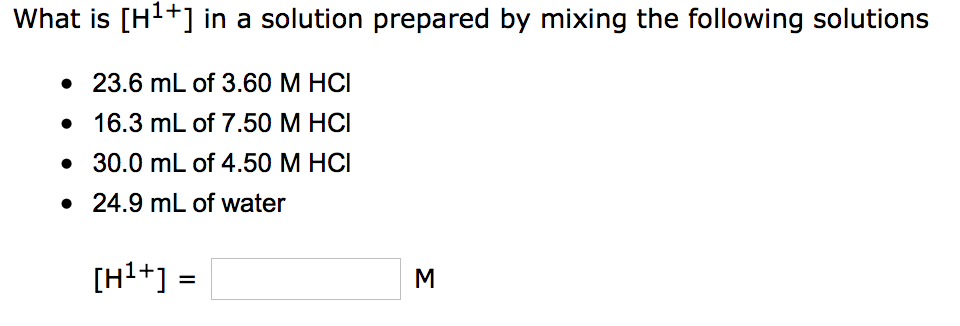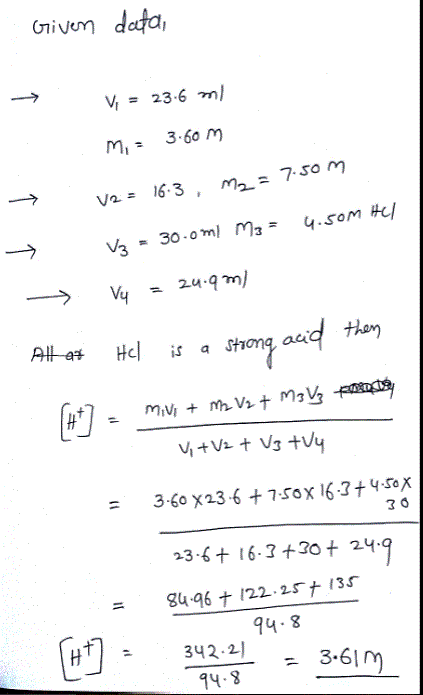# Question & Answer: What is [H^1+] in a solution prepared by mixing the following solutions 23.6 mL of 3.60 M…..What is [H^1+] in a solution prepared by mixing the following solutions 23.6 mL of 3.60 M HCl 16.3 mL of 7.50 MHCl 30.0 mL of 4.50 M HCl 24.9 mL of water [H^1+] = M